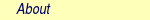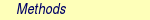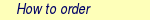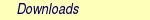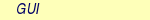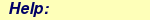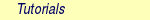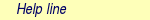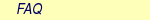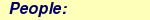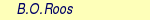MOLCAS manual:Next: 8.47 surfacehop Up: 8. Programs Previous: 8.45 single_aniso

Subsections

# 8.46 SlapAf

Provided with the first order derivative with respect to nuclear displacements the program is capable to optimize molecular structures with or without constraints for minima or transition states. This will be achieved with a quasi-Newton approach in combination with 2nd ranks updates of the approximate Hessian or with the use of a computed (analytic or numerical) Hessian. Note that if a computed Hessian is available on the RUNFILE then it will be used rather than the approximate Hessian generated by Slapaf. On completion of an optimization SlapAf will automatically execute a single energy evaluation.

## 8.46.1 Description

SlapAf has three different ways in selecting the basis for the displacements during the optimization. The first format require user input (not recommended), whereas the two other options are totally black-boxed. The formats are:

1. the old format as in MOLCAS-3, which is user specified. The internal coordinates are here represented as linear combination of internal coordinates (such as bonds, angles, torsions, out of plane angles, Cartesian coordinates and separation of centers of mass) and the linear combinations are totally defined by user input. This format does also require the user to specify the Hessian (default a diagonal matrix). This option allows for frozen internal coordinates.
2. the second format is an automatic option which employs the Cartesian eigenvectors of the approximative Hessian (generated by the Hessian model functional ).
3. the third format (this is the recommend and default) is an automatic option which utilizes linear combinations of some curvilinear coordinates (stretches, bends, and torsions). This implementation has two variations. The first can be viewed as the conventional use of non-redundant internal coordinates [148,149,150]. The second variation is a force constant weighted (FCW) redundant space (the HWRS option) version of the former implementation .
All three formats of internal coordinates can be used in combinations with constraints on the molecular parameters or other type of constraints as for example energy differences.

The displacements are symmetry adapted and any rotation and translation if present is deleted. The relaxation is symmetry preserving.

## 8.46.2 Dependencies

SlapAf depends on the results of ALASKA and also possibly on MCKINLEY and MCLR.

## 8.46.3 Files

### 8.46.3.1 Input files

Apart from the standard input file SlapAf will use the following input files.

 File Contents RUNFILE File for communication of auxiliary information. If a computed Hessian is available on this file it will be used rather than the approximate Hessian generated by Slapaf. RUNFILE2 File for communication of auxiliary information of the "ground state" in case of minimum energy cross point optimizations. RUNOLD File for communication of auxiliary information for reading an old Hessian matrix from a previous geometry optimization.

### 8.46.3.2 Output files

In addition to the standard output file SlapAf will use the following output files.

 File Contents RUNFILE File for communication of auxiliary information. RUNFILE2 File for communication of auxiliary information of the "ground state" in case of minimum energy cross point optimizations. MD_GEO Molden input file for geometry optimization analysis. MD_MEP Molden input file for minimum energy path (MEP). MD_SADDLE Molden input file for energy path (MEP) of a Saddle TS optimization. MD_IRC Molden input file for intrinsic reaction coordinate analysis of a TS. MD_FREQ Molden input file for harmonic frequency analysis. UNSYM ASCII file where all essential information, like geometry, Hessian normal modes and dipole derivatives are stored. STRUCTURE Output file with a statistics of geometry optimization convergence.

## 8.46.4 Input

SlapAf will as standard provided with an energy and a corresponding gradient update the geometry (optimize). Possible update methods include different quasi-Newton methods. The program will also provide for updates of the Hessian. The program has a number of different variable metric methods available for the Hessian update. This section describes the input to the SlapAf program.

This section describes the input to the SLAPAF program in the MOLCAS program system. The input starts with the program name

&SLAPAF

There are no compulsory keywords

Optional convergence control keywords
 Keyword Meaning ITERations Maximum number of iterations which will be allowed in the relaxation procedure. Default is 500 iterations, however, if environment variable MOLCAS_MAXITER has been exported by the user this is the assumed default value. THRShld Enter two real numbers which specifies the convergence criterion with respect to the energy change and the norm of the gradient. The defaults are 0.0 and 3.0D-4 au for Gaussian convergence criteria (which normally do not consider the energy change), and 1.0D-6 and 3.0D-4 for Baker criteria (see the BAKER keyword). BAKEr Activate convergence criteria according to Baker . Default is to use the convergence criteria as in the Gaussian program . MAXStep This keyword is followed by the value which defines the seed of largest change of the internal coordinates which will be accepted. A change which is larger is reduced to the max value. The value is dynamically modified each iterations. The default value is 0.3 au or rad. CNWEight Sets the maximum weight assigned to the fulfillment of the constraints, relative to the step taken in the complementary space for energy minimization. The step in the constraint space is truncated to be at most as large as the step in the minimization space, or half the maximum total step, whichever is larger, multiplied by this value. Default is 1.0. TOLErance Controls how strictly the constraints (if any) must be satisfied at convergence. The default value is very large, such that this criterion is always met, and only the gradient and maximum step (or energy difference) control convergence. If you set this keyword to some value, a constrained optimization will only converge if the maximum error in any constraint is lower than this number (in atomic units, and radians).

Optional coordinate selection keywords
 Keyword Meaning CARTesian Activate SlapAf to use the eigenvectors of the approximative Hessian expressed in Cartesian as the definition of the internal coordinates. The default is to use the FCW non-redundant internal coordinates. The Hessian will be modeled by the Hessian Model Functional. INTErnal This marks the start of the definition of the internal coordinates. This section is always ended by the keyword End of Internal. For a complete description of this keyword see the section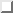. This option will also use a diagonal matrix as default for the Hessian matrix. The default is to use the FCW non-redundant internal coordinates. HWRS Use the force constant weighted (FCW) redundant space version of the nonredundant internal coordinates. This is the default. The Hessian will be modeled by the Hessian Model Functional. NOHWrs Disable the use of the force constant weighted redundant space version of the nonredundant internal coordinates. The default is to use the HWRS option. The Hessian will be modeled by the Hessian Model Functional. FUZZ When automatically generating the primitive internal coordinates, the system may end up in disconnected fragments, in which case additional bonds are defined between the fragments. This keyword controls how many inter-fragment bonds are added. Bonds are generated between the closest atoms of two fragments, and all pairs of atoms in separate fragments at a distance up to the specified value longer. The value can be followed with the unit BOHR or ANGSTROM. The default is 0.5 a.u.

Optional Hessian update keywords
 Keyword Meaning HUPDate Method used for updating the Hessian matrix. It must be one of: None -- No update is applied. BFGS -- Activate update according to Broyden-Fletcher-Goldfarb-Shanno. This is the default. MSP -- Activate the Murtagh-Sargent-Powell update according to Bofill . This update is preferred for the location of transition states. EU -- Activate the EU update according to Bofill . This update can be used for the location of transition states. TS-BFGS -- Activate the TS-BFGS update according to Bofill . This update can be used for the location of minima or transition states. UORDer Order the gradients and displacements vectors according to Schlegel prior to the update of the Hessian. Default is no reorder. WINDow Maximum number of previous iterations to include in the Hessian update. Default is 5.

Optional optimization procedure keywords
 Keyword Meaning NOLIne Disable line search. Default is to use line search for minima. RATIonal Activate geometry optimization using the restricted step Rational Functional optimization [156,157], this is the default. C1-Diis Activate geometry optimization using the C1-GDIIS method [158,159,160]. The default is to use the Rational Functional approach. C2-Diis Activate geometry optimization using the C2-GDIIS method . The default is to use the Rational Functional approach. DXDX This option is associated to the use of the C1- and C2-GDIIS procedures. This option will activate the computation of the so-called error matrix elements as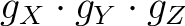, where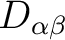is the displacement vector. DXG This option is associated to the use of the C1- and C2-GDIIS procedures. This option will activate the computation of the so-called error matrix elements as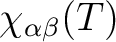, whereis the displacement vector and g is the gradient vector. GDX See above. GG This option is associated to the use of the C1- and C2-GDIIS procedures. This option will activate the computation of the so-called error matrix elements as, where g is the gradient vector. This is the default. NEWTon Activate geometry optimization using the standard quasi-Newton approach. The default is to use the Rational Functional approach. RS-P-rfo Activate RS-P-RFO  as default for TS-search. Default is RS-I-RFO. TS Keyword for optimization of transition states. This flag will activate the use of the mode following rational functional approach . The mode to follow can either be the one with the lowest eigenvalue (if positive it will be changed to a negative value) or by the eigenvector which index is specified by the MODE keyword (see below). The keyword will also activate the Murtagh-Sargent-Powell update of the Hessian and inactivate line search. This keyword will also enforce that the Hessian has the right index (i.e. one negative eigenvalue). MODE Specification of the Hessian eigenvector index, this mode will be followed by the mode following RF method for optimization of transition states. The keyword card is followed by a single card specifying the eigenvector index. FINDTS Enable a constrained optimization to release the constraints and locate a transition state if negative curvature is encountered and the gradient norm is below a specific threshold (see the GNRM option). Keyword TSCOnstraints should be used in combination with FINDTS. TSCOnstraints Specify constraints that will be active during the initial stage of an optimization with FINDTS. When negative curvature and low gradient are encountered, these constraints will be released and other constraints will remain active. If this block is not given in the input, all constraints will be released. The syntax of this keyword is exactly like normal constraints, and it must be ended with End of TSConstraints (see sectionbelow). GNRM Modify the gradient norm threshold associated with the FINDTS option. The actual threshold is specified on the subsequent line. The default value is 0.2. MEP-search Enable a minimum energy path (MEP) search. MEP is a valid synonym. NMEP Maximum number of points to find in a minimum energy path search or intrinsic reaction coordinate analysis. Synonym of NIRC. MEPStep The keyword is used to specify the step length done in the MEP search or IRC analysis. The step length can be followed with the unit BOHR or ANGSTROM. The default is 0.1 a.u. (in normalized mass-weighted coordinates). Synonym of IRCStep. MEPType Specifies what kind of constraint will be used for optimizing the points during the MEP search or IRC analysis. The possibilities are SPHERE, the default, which uses the Sphere constraint (each structure is at a given distance in coordinate space from the reference), or PLANE which uses the Transverse constraint (each structure is at a given distance from the hyperplane defined by the reference and the path direction). The reference structure changes at each step, according to the MEPAlgorithm keyword. Synonym of IRCType. MEPAlgorithm Selects the algorithm for a MEP search or IRC analysis. The possibilities are GS for the González-Schlegel algorithm, the default, or MB for the Müller-Brown algorithm. Synonym of IRCAlgorithm. REFErence The keyword is followed by a list of the symmetry unique coordinates (in au) of the origin of the hyper sphere. The default origin is the structure of the first iteration. GRADient of reference The keyword is followed by a list of the gradient vector components. This keyword is compulsory when using the Transverse kind of constraint. The optimization is performed in a space orthogonal to the given vector. IRC The keyword is used to perform an intrinsic reaction coordinate (IRC) analysis of a transition state structure. The analysis will follow the reaction path forward and backward until the energy increases. The keyword requires that the starting structure be that of a transition state and that the reaction vector be specified explicitly (check the keyword REACtion vector) or implicitly if it can be found on RUNOLD. Note that the user should not specify any explicit constraints! NIRC Maximum number of points to find in an intrinsic reaction coordinate analysis or minimum energy path search. Synonym of NMEP. IRCStep The keyword is used to specify the step length done in the IRC analysis or MEP search. The step length can be followed with the unit BOHR or ANGSTROM. The default is 0.1 a.u. (in normalized mass-weighted coordinates). Synonym of MEPStep. IRCType Specifies what kind of constraint will be used for optimizing the points during the IRC analysis or MEP search. The possibilities are SPHERE, the default, which uses the Sphere constraint (each structure is at a given distance in coordinate space from the reference), or PLANE which uses the Transverse constraint (each structure is at a given distance from the hyperplane defined by the reference and the path direction). The reference structure changes at each step, according to the IRCAlgorithm keyword. Synonym of MEPType IRCAlgorithm Selects the algorithm for a MEP search or IRC analysis. The possibilities are GS for the González-Schlegel algorithm, the default, or MB for the Müller-Brown algorithm. Synonym of MEPAlgorithm. REACtion vector The keyword is followed by the reaction vector specified as the Cartesian vector components on each of the symmetry unique atoms.

Optional force constant keywords
 Keyword Meaning OLDForce The Hessian matrix is read from the file RUNOLD. This Hessian is either an analytic or approximative Hessian updated by Slapaf. Note that for this option to work properly the type of internal coordinates must be the same! FCONstant Input of Hessian in internal coordinates. There are two different syntaxes. The keyword is followed by an entry with the number of elements which will be set (observe that the update will preserve that the elements Hij and Hji are equal). The next entries will contain the value and the indices of the elements to be replaced. The keyword if followed by the label Square or Triangular. The subsequent line specifies the rank of the Hessian. This is then followed by entries specifying the Hessian in square or lower triangular order. XFCOnstant Input of an external Hessian matrix in cartesian coordinates. The syntax is the same as for the FCONSTANT keyword. NUMErical This invokes as calculation of the force constant matrix by a two-point finite difference formula. The resulting force constant matrix is used for an analysis of the harmonic frequencies. Observe that in case of the use of internal coordinates defined as Cartesian coordinates that these has to be linear combinations which are free from translational and rotational components for the harmonic frequency analysis to be valid. Alternative: see keyword RowH in the section about Internal coordinates. CUBIc This invokes a calculation of the 2nd and the 3rd order force constant matrix by finite difference formula. DELTa This keyword is followed by a real number which defines the step length used in the finite differentiation. Default: 1.0D-2. PRFC The eigenvalues and eigenvectors of the Hessian matrix are printed. The internal coordinates definitions are also printed. RHIDden Define the hidden atoms selection radius in order to improve a QM/MM Hessian. It can be followed by Angstrom.

Optional miscellaneous keywords

 Keyword Meaning CTOF Coordinates TO Follow defines an internal coordinate whose values will be printed in the output during the optimization. Both the original and the new values will be printed. The keyword must be followed by the definition on the primitive coordinate. RTRN Maximum number of atoms for which bond lengths, angles and dihedral angles are listed, and the radius defining the maximum length of a bond follows. The latter is used as a threshold when printing out angles and dihedral angles. The length can be followed by Bohr or Angstrom which indicates the unit in which the length was specified, the default is Bohr. The default values are 15 and 3.0 au. THERmochemistry Request frequencies to be computed followed by an user specified thermochemical analysis. The keyword must be followed by different entries containing the Rotational Symmetry Number, the Pressure (in atm), and one entry per Temperature (in K) for which the thermochemistry will be calculated. The section is ended by the keyword End of PT. DISOtope Calculates frequencies modified for double isotopic substitution. TRACk Tries to follow electronic states during an optimization, by computing state overlaps with RASSI at each step. Root numbers selected with RlxRoot in RASSCF or with the EDiff'' constraint are only fixed in the first iteration, then the best-matching states are chosen. LASTenergy Specifies the quantum chemical method requested for the Last_Energy module (e.g., SCF, CASSCF, CASPT2, etc.) The keyword must be followed by the name of the module. Moreover, the EMIL command COPY needs to be used in the global input to provide a file named LASTEN, containing the input for the specified module. NOLAst energy Disables the call to the Last_Energy module when convergence is achieved.

Example: A complete set of input decks for a CASSCF geometry optimization. These are the input decks for the optimization of the enediyne molecule.

&GATEWAY
Title=  Enediyne
Coord=  $MOLCAS/Coord/enediyne.xyz Basis= ANO-L-VQZP Group= x z > DoWhile &SEWARD &SCF ITERATIONS= 30; Occupied= 9 8 2 1; Thresholds= 1.0d-8 1.0d-3 1.5d-3 0.2d-3; IVO &RASSCF Symmetry= 1; Spin= 1 NactEl= 12 0 0; Inactive= 7 7 0 0; Ras2= 3 3 3 3 Iterations= 50 50; CiRoot= 1 1; 1; Thrs= 1.0e-08 1.0e-05 1.0e-05 Lumorb &SLAPAF; Iterations= 20 > EndDo Example: Thermochemistry for an asymmetric top (Rotational Symmetry Number = 1), at 1.0 atm and 273.15, 298.15, 398.15 and 498.15 K. &SLAPAF; THERmochemistry= 1; 1.0; 273.15; 298.15; 398.15; 498.15; End of PT End of input ### 8.46.4.1 Definition of internal coordinates or constraints The input section defining the internal coordinates always start with the keyword Internal coordinates and the definition of the constraints starts with the keyword Constraints. Note that the latter is an input section for the GATEWAY module. The input is always sectioned into two parts where the first section defines a set of primitive internal coordinates and the second part defines the actual internal coordinates as any arbitrary linear combination of the primitive internal coordinates that was defined in the first section. In case of constraints the second part does also assign values to the constraints. In the first section we will refer to the atoms by their atom label (SEWARD will make sure that there is no redundancy). In case of symmetry one will have to augment the atom label with a symmetry operation in parenthesis in order to specify a symmetry related center. Note that the user only have to specify distinct internal coordinates (ALASKA will make the symmetry adaptation). In the specification below rLabel is a user defined label with no more than 8 (eight) characters. The specifications atom1, atom2, atom3, and atom4 are the unique atom labels as specified in the input to SEWARD. The primitive internal coordinates are defined as • rLabel = Bond atom1 atom2 -- a primitive internal coordinate rLabel is defined as the bond between center atom1 and atom2. • rLabel = Angle atom1 atom2 atom3 -- a primitive internal coordinate rLabel is defined as the angle between the bonds formed from connecting atom1 to atom2 and connecting atom2 to atom3. • rLabel = LAngle(1) atom1 atom2 atom3 -- a primitive internal coordinate rLabel is defined as the linear angle between the bonds formed from connecting atom1 to atom2 and connecting atom2 to atom3. To define the direction of the angle the following procedure is followed. 1. - the three centers are linear, 1. form a reference axis, R1, connecting atom1 and atom3, 2. compute the number of zero elements, nR, in the reference vector, 1. - nR=0, a first perpendicular direction to the reference axis is formed byfollowed by the projection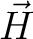The second perpendicular direction completes the right-handed system. 2. - nR=1, a first perpendicular direction to the reference axis is defined by setting the element in R2 corresponding to the zero entry in R1 to unity. The second perpendicular direction completes the right-handed system. 3. - nR=2, a first perpendicular direction to the reference axis is defined by setting the element corresponding to the first zero entry in R1 to unity. The second perpendicular direction completes the right-handed system. 2. - the three centers are nonlinear, the first perpendicular direction is the one which is in the plane formed by atoms atom1, atom2, and atom3. The second perpendicular direction is taken as the direction perpendicular to the same plane. The direction of the bend for LAngle(1) is taken in the direction of the first perpendicular direction, etc. • rLabel = LAngle(2) atom1 atom2 atom3 -- a primitive internal coordinate rLabel is defined as the linear angle between the bonds formed from connecting atom1 to atom2 and connecting atom2 to atom3. The definition of the perpendicular directions is as described above. The direction of the bend for LAngle(2) is taken in the direction of the second perpendicular direction. • rLabel = Dihedral atom1 atom2 atom3 atom4 -- a primitive internal coordinate rLabel is defined as the angle between the planes formed of atom1, atom2 and atom3, and atom2, atom3 and atom4, respectively. • rLabel = OutOfP atom1 atom2 atom3 atom4 -- a primitive internal coordinate rLabel is defined as the angle between the plane formed by atom2, atom3, and atom4 and the bond formed by connecting atom1 and atom4. • rLabel = Dissoc (n1+n2) atom1 atom2 atom3 ... atomN -- a primitive internal coordinate rLabel is defined as the distance between the center of masses of two sets of centers. The first center has n1 members and the second has n2. The input contains the labels of the atoms of the first group followed immediately by the labels of the second group. This option is not available for constraints. • rLabel = Cartesian i atom1 -- a primitive internal coordinate rLabel is defined as the pure Cartesian displacement of the center labeled atom1. The label i is selected to x, y, or z to give the appropriate component. • rLabel = Ediff [i j] -- the energy difference between states i and j (if provided, the brackets indicate they are optional, do not include the brackets). If i and j are not provided, the difference is between the current'' state and the state provided on RUNFILE2. This is only used in constrained optimization in which crossings or conical intersections are located. If this constraint is used, the average energy of the two states will be optimized, subject to the constraint. If the value is 0.0 and the spin and spatial symmetry of both states is the same, a conical intersection will be searched. In this case, SLAPAF will request an analytical calculation of the nonadiabatic coupling vector, if available. If it is not available, or if RUNFILE2 is being used (i and j not provided), the branching space update method of Maeda et al. will be used . • rLabel = Sphere -- the radius of the hypersphere defined by two different molecular structures (the origin is the first structure) in relative mass-weighted coordinates. This is only used in constrained optimization in which minimum reaction paths (MEP) or intrinsic reaction coordinate (IRC) paths are followed. The units of the radius is in mass-weighted coordinates divided with the square root of the total mass of the molecule. • rLabel = Transverse -- a level of orthogonality''. This is used to perform an optimization in a space orthogonal to a given vector. Recommended value 0.0. Requires usage of GRAD keyword. • rLabel = Fragment atom1 atom2 atom3 atom4 -- a dummy internal coordinate rLabel is defined. This translates to that a set of internal coordinates are generated automatically according to a standard Z-matrix format to define all degrees of freedom of the fragment defined by the list of atoms on the same line. These internal coordinates will be automatically fixed in the geometry optimizations to the values of starting structure. Note, the values of these do not need to be explicitly defined and set in the Values section. Note, too, that the generation of the internal coordinates is done according to the order in which atom1, atom2, etc. are given; for some systems, especially with linear angles, it may be preferable to define the coordinates manually. The second section starts with the label Vary or in the case of constraints with the label Values. In case of a definition of internal coordinates in this section the user specifies all symmetric internal coordinates excluding translation and rotation using a list of expressions like label = f1 rLabel1 + f2 rLabel2 + .... which defines an internal coordinate label as the linear combination of the primitive internal coordinates rLabel1, rLabel2, ... with the coefficients f1, f2, ..., respectively. If the internal coordinate just corresponds to the primitive internal coordinate, the same label can be used label If some internal coordinates are chosen to be fixed they should be defined after the label Fix. The fixed internal coordinate are defined with expressions as in the section Vary. Observe: using expression can introduce linear dependence and/or undefined nuclear coordinates, so use with care. For the internal coordinates defined after Vary (and Fix, if present) a numerical estimation of rows and columns of the hessian matrix can be performed. The label of internal coordinates (max 10) must be specified after keyword RowH. Keywords NUMErical and RowH are mutually exclusive. In case of a definition of constraints the sections contains either a direct reference to a rLabel as in rLabel = rValue [Angstrom,Degrees] [Soft,Hard] [Phantom] or one can also use expressions like f1 rLabel1 +/- f2 rLabel2 +/- .... = Value [Angstrom,Degrees] [Soft,Hard] [Phantom] where rValue is the desired value of the constraint in au or rad, or in angstrom or degrees if the corresponding keyword is added. The Hard'' and Soft'' keywords are only meaningful for numerical differentiation: the coordinates corresponding to soft constraints are differentiated, those of hard constraints are not . By default almost all constraints are hard, only constraints of the type Sphere'', Transverse'' and Ediff'' default to soft. The Hard'' and Soft'' keywords override the default. When using constraints in combination with the FINDTS keyword, one should use soft constraints, at least for the constraint most similar to the expected reaction vector. Constraints defined in TSCOnstraints (recommended) are automatically considered soft. The Phantom'' modifier can be used to ignore a constraint in the optimization. A phantom constraint will only be considered for numerical differentiation. Phantom constraints are useful in combination with the KEEPOldGradient keyword of ALASKA. Using NGEXclude in GATEWAY is equivalent to phantom constraints, and it is the preferred way to set up composite gradients . Alternatively, if the current value of an internal coordinate is to be used, i.e. no change is to be allowed (frozen), this is expressed as rLabel = FIX [Soft,Hard] [Phantom] Note that a coordinate of type Fragment'' does not need to appear in the Values section, but if it does it must be assigned the value FIX''. Example: A definition of user specified internal coordinates of benzene. The molecule is in D6h and since MOLCAS only uses up to D2h the Fix option is used to constrain the relaxation to the higher point group. Observe that this will only restrict the nuclear coordinates to D6h. The electronic wavefunction, however, can have lower symmetry. Internal coordinates r1 = Bond C1 C2 r2 = Bond C1 H1 r3 = Bond C2 H2 r4 = Bond C2 C2(x) f1 = Angle H1 C1 C2 f2 = Angle H2 C2 C1 Vary a = 1.0 r1 + 1.0 r4 b = 1.0 r2 + 1.0 r3 c = 1.0 f1 + 1.0 f2 Fix a = 1.0 r1 + -1.0 r4 b = 1.0 r2 + -1.0 r3 c = 1.0 f1 + -1.0 f2 End of Internal Example: A input for the optimization of water constraining the structure to be linear at convergence. &GATEWAY Title= H2O geom optim, using the ANO-S basis set. Coord=$MOLCAS/Coord/Water.xyz
Basis=ANO-S-VDZ
Group=  c1
Constraints
a1  =  langle(1)  H2  O1  H3
Values
a1  =  179.99  degrees
End  of  Constraints
>>>  DO  WHILE  <<<
&SEWARD;  &SCF
&SLAPAF
>>>  END  DO  <<<
Example: A complete set of input decks for a UHF transition structure geometry optimization of an identity hydrogen transfer reaction (HO + H2O -> H2O + OH).

&GATEWAY
ZMAT
O.STO-3G....
H.STO-3G....

H1
Z2  1  1.0
O3  1  1.15  2  92.
O4  1  1.15  2  92.  3  180.
H5  3  0.98  4  105.4  2  120.
H6  4  0.98  3  105.4  2  120.

>>>  DO  WHILE  <<<
&SEWARD;
&SCF;  UHF
&SLAPAF;  TS;  PRFC
Internal
bOO4  =  Bond  O3  O4
bOH5  =  Bond  H5  O3
bOH6  =  Bond  H6  O4
bOH1  =  Bond  O3  H1
aOOH5  =  Angle  O4  O3  H5
aOOH6  =  Angle  O3  O4  H6
aHOH1  =  Angle  H5  O3  H1
dH6  =  Dihedral  H6  O4  O3  H5
dH1  =  Dihedral  O4  H5  O3  H1
Vary;  bOO4;  bOH5;  bOH6;  bOH1;  aOOH5;  aOOH6;  aHOH1;  dH6;  dH1
RowH;  bOH1
End  of  Internal

>>>  ENDDO  <<<

Example: Optimization of a minimum energy conical intersection point, using automatic calculation of analytical gradients and nonadiabatic coupling.

&GATEWAY
Coord  =  acrolein.xyz
Basis  =  cc-pVDZ
Group  =  NoSymm
Constraints
a  =  Ediff  1  2
Values
a  =  0.0
End  of  constraints

>>>  DoWhile

&SEWARD

>>>  If  (iter  =  1)
&SCF
&MBPT2
PrPt
>>>  EndIf

&RASSCF
FileOrb  =  \$Project.MP2Orb
Charge  =  0
NActEl  =  6  0  0
RAS2  =  5
CIRoot  =  4  4  1

&SLAPAF

>>>  EndDo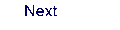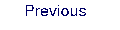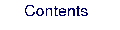Next: 8.47 surfacehop Up: 8. Programs Previous: 8.45 single_aniso